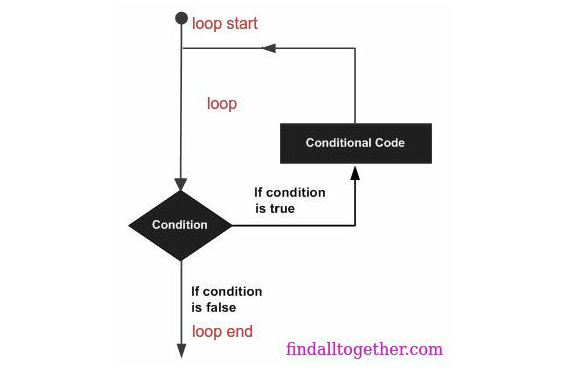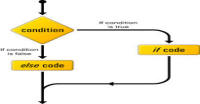# Loops in python

If we have to do same thing again and again then we have not to write code for each time. We will write code only once and will use that code rapidly. We do this by using loops. In Python we have two types of loops: while loop and for loop. We will start with a simple example. Lets assume we want to print numbers from 1 to 100. We already know that we have to use print() function for it.

### While Loop:

In while loop, we have to define a condition which will be checked every time and until that condition is not satisfied our code will be run. In above example, we have our number is less than 101 so we will check that we print until we have not reach at 101. Lets check this example:

```#programe to print numbers from 1 to 100
# initializing variable
number = 1
#checking condition
while number < 101:
#writing repeating code
print(number)
#changing condition
number = number + 1
```

It is clear from code that we have to write condition which are same as conditions in conditional statements. Then we have to write code which we want to execute again and again. And at last change the condition which will control the code.

NOTE: We can also write condition before code (inside loop), it will not effect the program.

### For loop:

It is also similar to while loop. Above example can also be written as:

```#programe to print numbers from 1 to 100
for number in range(100):
#writing repeating code
print(number+1)
```

Here we are using for loop instead of while loop. range function of python is a set, i.e. it contains values from 0 to 99 (100 values). Every time number is initialized with next value of range. Because number is starting from 0 so we are printing number + 1.

How is it similar to while loop?

In while loop, first we check value / condition here also we check condition that number is in range. Similarly here also we are writing code inside loop and changing value of number is automatically handled by range. Look at this example:

```#printing the list
# we are initializing a list
list = ['name','harish','age',20,'number',10]

# in for loop we are using list instead of range
for temp in list:
# priting each value of list
print(temp)
```

Now you should understand that inside for loop statements, we have two variables:

1. One iterable variable like list, luple, set or dictionary. This variable will assign value to another variable.
2. Another variable which will take value ( in above example: temp)

### Loops with else statement:

If you are coming from other programming language like C, C++, Java or PHP then you will be surprised that in python loops has also else statements. It is slightly different for if else statement. In loop, we check the condition if condition satisfies then code of loop will be executed otherwise code of else statement will be executed. It is obvious that code of else statement in loops will be execute every time after loop.

```#example of else statement
# we are initializing a list
list = ['name','harish','age',20,'number',10]

# in for loop we are using list instead of range
for temp in list:
# priting each value of list
print(temp)
else:
print('list finished')

condition = False

while condition:
print('condition true')
else:
print('condition false')
```

Output is:

```name
harish
age
20
number
10
list finished
condition false
```

### Nested loops:

Same as conditional statements, you can write loop inside loop.

```#this is nested loop

for number in range(10):
while number < 5:
print(number)
number = number +1;
# another code inside for loop
print('outside while loop')
```

Output is:

```0
1
2
3
4
outside while loop
1
2
3
4
outside while loop
2
3
4
outside while loop
3
4
outside while loop
4
outside while loop
outside while loop
outside while loop
outside while loop
outside while loop
outside while loop
```

### Break Statement:

Assume a loop is in execution and we want to stop it then we use break statement to come out the loop. After breaking code after break statement will not be executed.

```# break statement

number = 1
# infinite loop
while True:
print('this is while loop')
# increasing number by one
number += 1
if number == 5:
# if number is 5 then break loop
break
print('loop does not break till now')
```

In this example, last print statement 'loop does not break till now will not be printed when number is 5.

```this is while loop
loop does not break till now
this is while loop
loop does not break till now
this is while loop
loop does not break till now
this is while loop
```

NOTE: If you are coming from such a programming language which has do while loop then above example is of do while loop in python because code inside the loop will always execute atleast one time.

### Continue Statements:

It is used to skip some code from execution.

```# continue statement

# for loop from 0 to 9
for number in range(10):
if number == 5:
continue
print(number)
```

In this example, we are skipping 5 from printing. Output is:

```0
1
2
3
4
6
7
8
9
```

#### Click on banner below to Learn: PHP with Laravel for beginners - Become a Master in LaravelHarish, a technical core team member at www.lyflink.com with five year experience in full stack web and mobile development, spends most of his time on coding, reading, analysing and curiously following businesses environments. He is a non-graduate alumni from IIT Roorkee, Computer Science and frequently writes on both technical and business topics.

### Related ArticlesPHP stands for PHP: Hypertext Preprocessor. PHP is known as a server side scripting and interpreter language for web ...
##### Introduction to PHPIn this tutorial, we will study about conditional statements (if else statements and switch statements). These statem...
##### Conditional statements in phpIn this tutorial, we will study about strings and operations upon strings. Due to long length of this section, I have...

### The Complete Web Developer Course - Build 25 Websites